# Apéry numbers

(Redirected from Apery numbers)
The Apéry numbers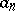,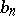are defined by the finite sumsfor every integer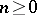. They were introduced in 1978 by R. Apéry in his highly remarkable irrationality proofs of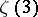and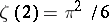, respectively. In the case of, Apéry showed that there exists a sequence of rational numberswith denominator dividingsuch that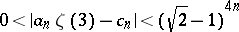for all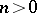. Together with the fact that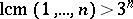, this implies the irrationality of. For a very lively and amusing account of Apéry's discovery, see [a4]. In 1979 F. Beukers [a1] gave a very short irrationality proof of, motivated by the shape of the Apéry numbers. Despite much efforts by many people there is no generalization to an irrationality proof of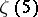so far (2001).
T. Rival [a5] proved the very surprising result that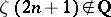for infinitely many.
It did not take long before people noticed a large number of interesting congruence properties of Apéry numbers. For example,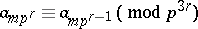for all positive integers,and all prime numbers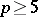. Another congruence isfor all prime numbers. Here,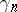denotes the coefficient of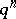in the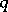-expansion of a modular cusp form. For more details see [a2], [a3].